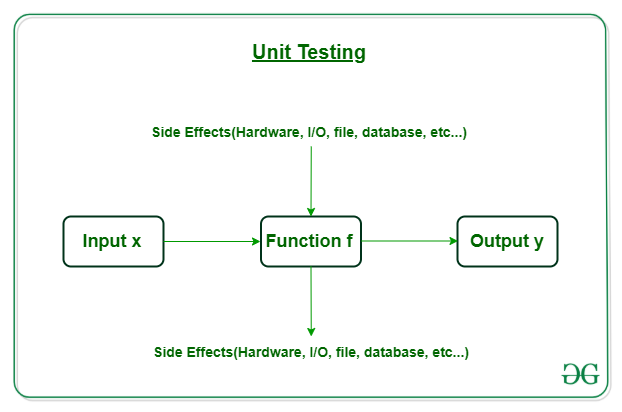# Unit Testing in R Programming

The unit test basically is small functions that test and help to write robust code. From a robust code we mean a code which will not break easily upon changes, can be refactored simply, can be extended without breaking the rest, and can be tested with ease. Unit tests are of great use when it comes to dynamically typed script languages as there is no assistance from a compiler showing you places where functions could be called with invalid arguments.

#### How Does Unit Testing Work?What does a simple function do, it takes some input x and returns an output y. In unit testing, we verify the preciseness and correctness of the function is returning the expected value of y for a specific value of x when calling the function. Generally, different (x, y) pairs are tested. What if our code has side effects e.g reading/writing of files, access to some database, etc. then how does a unit test work. In such scenarios, the preparation of the test is more complex. It could comprise just a bunch of mock objects of functions for simulating access to a database. That is influencing the programming style for which abstraction layers might become necessary. In some cases, input files need to be generated before executing the test, and output files are to be checked after the test. The basic idea behind unit testing is simple, you write a script that automatically evaluates pieces of your code and checks it against expected behavior. Now let us see some examples for a better understanding of what actually unit testing means and how it works.

#### Implementation in R

In R programming testthat package helps us to implement unit testing in our codes. To install testthat package one just need to run the following code in his R console.

```if (!require(testthat)) install.packages('testthat')
```

testthat uses test_that() function to create a test and uses expectations for unit testing of code. An expectation allows us to assert that the values returned by a function match the ones we should get.

```test_that("Message to be displayed",
{ expect_equal(function_f(input x), expected_output_y)
expect_equivalent(function_f(input x), expected_output_y)
expect_identical(function_f(input x), expected_output_y)
.
.
.
})
```

There are more than 20 expectations in the testthat package.

Example: Define a function factorial that takes a numeric value n and returns its factorial.

## R

 `# create a recursive program that  ` `# calculates the factorial of number n ` `factorial <- ``function``(n) ` `{ ` `  ``if``(n == 0) ` `  ``{ ` `    ``return``(1) ` `  ``} ` `  ``else` `  ``{ ` `    ``return``(n * ``factorial``(n - 2)) ` `  ``} ` `}`

Now, let’s perform unit testing on our function factorial and test its accuracy and debug our program.

## R

 `# import testthat package ` `library``(testthat) ` ` `  `# use expect_that to create tests ` `expect_that ` `( ` `  ``"Factorial of number \$n"``, ` `  ``{ ` `    ``expect_equal``(``factorial``(5), 120) ` `    ``expect_identical``(``factorial``(2), 2) ` `    ``expect_equal``(``factorial``(8), 40320) ` `  ``} ` `)`

Output:

```Error: Test failed: 'Factorial computed correctly'
* factorial(5) not equal to 120.
1/1 mismatches
 15 - 120 == -105
* factorial(8) not equal to 40320.
1/1 mismatches
 384 - 40320 == -39936
```

The test gives an error, it means that our function does not return the desired results. We knowingly wrote our code wrong. In the factorial function, the recursive approach we used has an error. Let us eradicate that error and test our function one more time.

## R

 `# create a recursive program that ` `# calculates the factorial of number n ` `factorial <- ``function``(n) ` `{ ` `  ``if``(n == 0) ` `  ``{ ` `    ``return``(1) ` `  ``} ` `  ``else` `  ``{ ` `    ``# notice we used (n-2) instead ` `    ``# of (n-1) in our previous code ` `    ``return``(n * ``factorial``(n - 1)) ` `  ``} ` `} ` ` `  `# import testthat package ` `library``(testthat) ` ` `  `# use expect_that to create tests ` `expect_that ` `( ` `  ``"Factorial of number \$n"``, ` `  ``{ ` `    ``expect_equal``(``factorial``(5), 120) ` `    ``expect_identical``(``factorial``(2), 2) ` `    ``expect_equal``(``factorial``(8), 40320) ` `  ``} ` `)`

```# no error
```

Note: If your source code and packages are not in the same directory you have to run a line of code with function source( ) to run tests.

```source("your_file_path")  # This is only needed if your project is not a package
```

It is really important to organize your files and tests. We should have a folder named R with all the R code files, and one folder named tests/testthat, where all the test scripts will live. From the R console, you can run all tests in a file with

```test_file("./path/to/file")
```

And all tests in a folder with

```test_dir("./path/to/folder")
```

Both the above-mentioned function accept a special parameter reporter which has several options to offer which are

• progress  it is the default value
• minimal   for a minimal report
• location   shows the file, line, and column of the test run (failed or otherwise),
• debug      allows you to debug interactively a failing test and more.
```test_dir("./path/to/folder", reporter=c("minimal", "location"))
```

Unit testing is necessary if you want a bug free, well-formed code.

My Personal Notes arrow_drop_upCheck out this Author's contributed articles.

If you like GeeksforGeeks and would like to contribute, you can also write an article using contribute.geeksforgeeks.org or mail your article to contribute@geeksforgeeks.org. See your article appearing on the GeeksforGeeks main page and help other Geeks.

Please Improve this article if you find anything incorrect by clicking on the "Improve Article" button below.

Article Tags :

1

Please write to us at contribute@geeksforgeeks.org to report any issue with the above content.